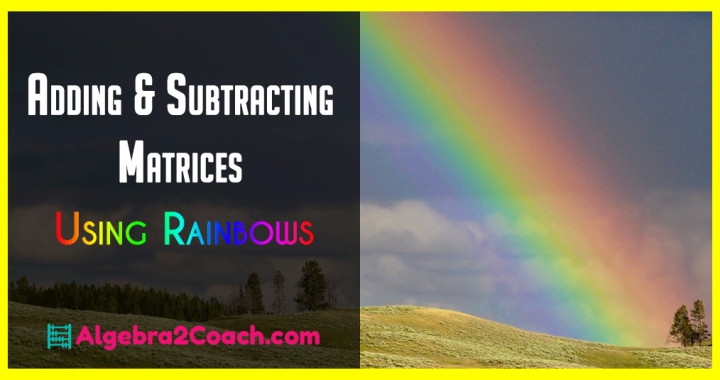# Adding and Subtracting Matrices – Using Rainbows

 Would You Rather Listen to the Lesson?This is the most important lesson in the Unit on Matrices! The easiest way to teach adding and subtracting matrices is by color coding.

## Let's Start Adding and Subtracting Matrices

I start with adding two 2x3 matrices. The reason I do not start with 2x2 matrices is because it is easier to show them that the matrices must be the same size to add or subtract them.

The other reason I use 2x3 matrices is because that means there will be 6 numbers inside each and there are 6 colors to the rainbow (I know that's debatable but for the sake of the lesson go with it).

Red - Orange - Yellow - Green - Blue - Purple

Each number from the first matrix gets matched with its Corresponding number in the second matrix. Then they are added or subtracted. I do this with a group activity.

Cards like the ones below are passed out to small groups and they find the solutions. The rest of the activity is available if you are a Algebra 2 Teacher Community Member: Click here to Join Now!

Here are two cards I use to introduce the lesson...

## Here is your free content for this lesson!

### Adding and Subtracting Matrices Word Docs & PowerPoints

To gain access to our editable content Join the Algebra 2 Teacher Community!
Here you will find hundreds of lessons, a community of teachers for support, and materials that are always up to date with the latest standards.

### Adding and Subtracting Matrices PDFs

4-2 Assignment Teacher Edition - (Members Only)

4-2 Bell Work Teacher Edition - (Members Only)

4-2 Exit Quiz Teacher Edition - (Members Only)

4-2 Guided Notes Teacher Edition - (Members Only)

4-2 Lesson Plan - Adding and Subtracting Matrices (Members Only)

4-2 Online Activities (Members Only)